Mathematics
Easy

Question

# The relationship between speed(s), distance(d) and time(t) is given by s =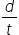. Find the value of s, d = 135 m and t =10 m

##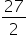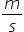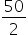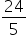None of the aboveHint:

## The correct answer is:### The given expression is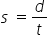There are three variables here. The values of two variables are given. We have to use the relation between them and find the third value.The values of variables is as follows:d = 135mt = 10sWe have to substitute these values in the above expression and we will get the value of ‘s’.To substitute the values we will replace the variables by their respective values.So we getTherefore, the value of speed is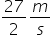.   So option (a) is the right option.

We have to be careful about the operations because of the variables. We have to follow the proper procedure for performing the operations. The variables take different values under different conditions. So we have to be careful while substituting the values. The main part of this type of question is just replacing the variables by their values and performing the operation.

### Related Questions to study#### With Turito Foundation.#### Get an Expert Advice From Turito.